## Friday, July 31, 2009

### 475

475 = 52 x 19.

475 as a square that is composed of overlapping squares of smaller numbers: 4752 = 225625.

475 is the smallest of three consecutive numbers, each one divisible by a square other than 1 (476 is divisible by 4 and 477 is divisible by 9).

475 has the same last two digits as 4753: 4753 = 107171875.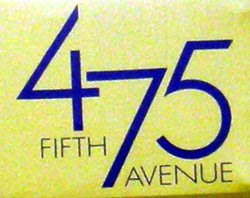475 Ocllo is an asteroid discovered in 1901.

## Thursday, July 30, 2009

### 356

356 = 22 x 89.

356356 + 356! + 356*356 + 356/356 - (356 + 356) is a 909-digit prime number.

356 = 26 + 44 + 62.

356 has a representation as a sum of two squares: 356 = 102 + 162.The
Porsche 356 was the company's first production automobile.

Source:
Prime Curios!

## Wednesday, July 29, 2009

### 229

229 is a prime number.

227 and 229 form a twin prime pair.

229 has a representation as a sum of two squares: 229 = 22 + 152.

229 is the hypotenuse of a primitive Pythagorean triple: 2292 = 602 + 2212.

The sum of the first
229 primes divides the product of the first 229 primes.

229 is the smallest prime that remains prime when added to its reversal: 229 + 922 = 1151.

229 is the difference between 33 and 44.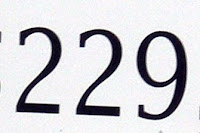229 is the highest you can score in a standard game of bowling if your score in each of the ten frames is required to be a prime number.

Source:
Prime Curios!

## Tuesday, July 28, 2009

### 127

127 is a prime number.

127 is a Mersenne number: 27 – 1 = 127. It is the largest Mersenne prime with all different digits.

127 = 20 + 21 + 22 + 23 + 24 + 25 + 26.

127 can be expressed as the sum of factorials of the first three odd numbers: 1! + 3! + 5! = 127.127 is the number of prime days in a leap year.

Source:
Prime Curios!

## Monday, July 27, 2009

### 24

24 = 23 x 3.

24 has a unique representation as a sum of three squares: 24 = 22 + 22 + 42.

Factorial 4 = 4! = 4 x 3 x 2 x 1 =
24.

24 is the smallest number with three representations as a sum of two distinct primes: 24 = 5 + 19 = 7 + 17 = 11 + 13.

24 is the largest number divisible by all numbers less than its square root.

24 is 44 in base 5.A cuboctahedron is an Archimedean solid with
24 edges.Source:
Number Gossip

## Friday, July 24, 2009

### 210

210 = 2 x 3 x 5 x 7 (the first four primes).

210 is both a triangular number and a pentagonal number. It is the smallest such number (other than 1); the next smallest is 40,755.

210 is the smallest area of two different primitive Pythagorean triangles: 20, 21, 29 and 12, 35, 37.

The primes
47, 257, 467, 677, 887, 1097, and 1307 constitute a progression of seven terms with a common difference of 210.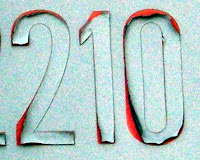210 Isabella is a large, dark main-belt asteroid discovered in 1879.

Source:
The Penguin Dictionary of Curious and Interesting Numbers, rev. ed., by David Wells.

## Thursday, July 23, 2009

### 250

250 = 2 x 53.

250 has two representations as a sum of two squares: 250 = 52 + 152 = 92 + 132.

250 is the smallest multidigit number such that the sum of the squares of its prime factors equals the sum of the squares of its digits.

1
3 + 33 + 33 = 55, 53 + 53 = 250 and 23 + 53 + 03 = 133.

250 is 505 in base 7.In the year
250, Diophantus writes Arithmetica, the first systematic treatise on algebra.

Source:
The Penguin Dictionary of Curious and Interesting Numbers, rev. ed., by David Wells.

## Wednesday, July 22, 2009

### 281

281 is a prime number. It is also a Sophie Germain prime because 2 x 281 + 1 = 563 is prime.

281 has a representation as a sum of two squares: 281 = 52 + 162.

281 and 283 form a twin prime pair.

281 is the hypotenuse of a primitive Pythagorean triple: 2812 = 1602 + 2312.

The final repeated digit-products of consecutive integers
281, 282, and 283 are all 6: 2 x 8 x 1 = 16, 1 x 6 = 6; 2 x 8 x 2 = 32, 3 x 2 = 6; 2 x 8 x 3 = 48, 4 x 8 = 32, 3 x 2 = 6. There are no four consecutive integers with the same final digit-product less than 6 x 24 x 108.

281 is the sum of the first 14 primes (up to 43). If we add consecutive primes up to 281, the total is also a prime (7699). There are no other such primes with this behavior between 281 and 7699.281 is a telephone area code for Houston, Texas.

Source:
The Penguin Dictionary of Curious and Interesting Numbers, rev. ed., by David Wells.

## Tuesday, July 21, 2009

### 1331

1331 is a perfect cube.

1331
= 113 = (13 – 3 + 1)3 = (1 + 3 x 3 – 1)3.

1331 is that smallest cube (other than 1) containing only odd digits. It is also the smallest cube having every digit it contains exactly twice

1331 = 192 + 212 + 232.

1331 = 113 = 439 + 443 + 449 is the smallest solution of three consecutive primes summing to the third power of a prime.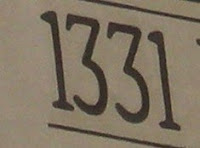A
partial solar eclipse occurred on July 5 in the year 1331.

Source: The Penguin Dictionary of Curious and Interesting Numbers, rev. ed., by David Wells.

## Monday, July 20, 2009

### 1634

1634 = 2 x 19 x 43.

1634 is the smallest number (except 1) that is the sum of the fourth powers of its digits: 1634 = 14 + 64+ 34 + 44. The other four-digit numbers that are the sums of the fourth powers of their digits are 8208 and 9474. A number that is the sum of the nth powers of its digits is called a narcissistic number.

1634 and 1635 have the same sum of divisors: 1 + 2 + 19 + 38 + 43 + 86 + 817 + 1634 = 1 + 3 + 5 + 15 + 109 + 327 + 545 + 1635. They also both have the same number of divisors.

1634, 1635, and 1636 are each the product of exactly three primes.

In base 5, 1634 has five distinct digits: 23014.In the year
1634, the first settlers arrived in St. Mary's City, Md., to form the fourth permanent settlement in British North America.

Source:
The Penguin Dictionary of Curious and Interesting Numbers, rev. ed., by David Wells.

## Friday, July 17, 2009

### 545

545 = 5 x 109.

545 is a centered square number.

545 is the smallest palindrome resulting from the sum of an ascending sequence of consecutive primes with a descending sequence of the same primes as exponents: 27 + 35 + 53 + 72 = 545.

1*1! + 2*2! + 3*3! + . . . + 545*545! is a titanic prime.

545 has a base 3 representation (202012) that begins with its base 4 representation (20201).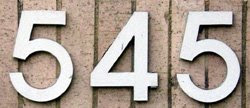545 people are said to govern the United States: 435 representatives, 100 senators, 1 president, and 9 justices of the Supreme Court.

## Thursday, July 16, 2009

### 444

444 = 22 x 3 x 37.

444444 + 5 is prime. Note that 444 is the only known number m such that mm + 5 is prime.

444 = (41 + 41+ 41) + (42 + 42 + 42) + (43 + 43 + 43) + (43 + 43 + 43).

In base 6, 444 is 2020.St. Patrick founded the Irish city of Armagh in the year 444.

Source: Prime Curios!

## Wednesday, July 15, 2009

### 325

325 = 52 x 13.

325 is the 25th triangular number (the sum of the first 25 integers): 1 + 2 + 3 + . . . + 25 = 325.

In base 2,
325 is the palindromic number 101000101; in base 4, it is 11011; and in base 8, it is 505.

325 is the smallest number to be the sum of two squares in three different ways: 325 = 12 + 182 = 62 + 172 = 102 + 152.

325 is the hypotenuse of two primitive Pythagorean triples: 3252 = 362 + 3232 = 2042 + 2532.Currently undergoing restoration, the
USS LST-325, launched in 1942, participated in the invasion of Sicily in 1943 and in June 1944 landed at Omaha Beach.

## Tuesday, July 14, 2009

### 221

221 = 13 x 17.

221 = 2*3*5*7 + 11 = 13*17. Note the consecutive use of the first seven primes.

221 has two representations as a sum of two squares: 221 = 52 + 142 = 102 + 112.

221 is the hypotenuse of two primitive Pythagorean triples: 2212 = 212 + 2202 = 1402 + 1712.221 is a centered square number.221b, Baker Street, London, England is the address of fictional detective Sherlock Holmes.

Source:
Prime Curios!

## Monday, July 13, 2009

### 110

110 = 2 x 5 x 11.

110 is the product of a prime and the previous number: 110 = 11 x 10.

110 is the sum of three consecutive squares: 110 = 52 + 62 + 72.

144
5 = 1335 + 1105 + 845 + 275: The first counterexample to disprove Euler’s conjecture that it always requires at least n terms (of nth power) to sum to an nth power, nontrivially.

110 is a Niven (or Harshad) number because it is divisible by the sum of its digits.110 hertz is a frequency of the musical note A.

Source:
Numeropedia

## Friday, July 10, 2009

### 98

98 = 2 x 72

98 is the smallest number that starts a sequence of three consecutive numbers with at least three prime divisors.

98 is the smallest number with the property that its first five multiples contain the digit 9: 98, 196, 294, 392, 490.

98 = 14 + 24 + 34.

98 has a representation as a sum of two squares: 98 = 72 + 72.

98 is 77 in base 13.98 is the atomic number of the radioactive element Californium.

Source: Number Gossip

## Thursday, July 9, 2009

### 12,837,064

12,837,064 = 23 x 631 x 2543 (via WolframAlpha)

12,837,064 is the number of decimal digits in the 47th known Mersenne prime, discovered April 12, 2009.

This prime is at present the second largest known prime number, a mere 141,125 digits smaller than the record-breaking Mersenne prime found last August.

Source: Great Internet Mersenne Prime Search

## Wednesday, July 8, 2009

### 993

993 = 3 x 331.

993 arises in the sequence generated by starting with 1, then repeatedly reversing the digits and adding 4: 1, 5, 9, 13, 35, 57, . . . , 989, 993, 403, . . .

993 is the sum of three consecutive pentagonal numbers: 993 = 287 + 330 + 376.

993 is a number n that is a product of two primes j and k such that n + j + k and n - j - k are both primes: 993 + 3 + 331 = 1327 and 993 - 3 - 331 = 659.993 is the number of paraffins with 8 carbon atoms.

The fantasy role-playing game Dragon Warriors was set in a fantasy world called "Legend," modeled on medieval Europe in roughly 993 AD.

Source: Wikipedia

## Tuesday, July 7, 2009

### 892

892 = 22 x 223.

892 is the smallest integer ratio of a 13-digit number to its product of digits.

892 is a number that cannot be written as a sum of three squares.

892 is a number such that the sum of its base 3 digits (1020001) equals the sum of its base 9 digits (1201).NGC 892 is a galaxy in the constellation Cetus.

## Monday, July 6, 2009

### 730

730 = 2 x 5 x 73.

730 is 1000001 in base 3 and 1001 in base 9.

730 is the number of connected bipartite graphs with 9 vertices.

730 has two representations as a sum of two squares: 730 = 12 + 272 = 172 + 212.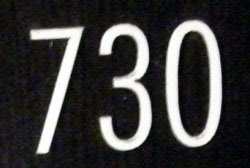In the Urban Dictionary, 730 refers to someone who is crazy.

## Thursday, July 2, 2009

### 525

525 = 3 x 52 x 7.

525 in base 6 is 2233.

525 is a hexagonal pyramidal number (greengrocer's number).

525 is a number with exactly 12 divisors: 1, 3, 5, 7, 15, 21, 25, 35, 75, 105, 175, 525.Dionysius Exiguus produced his tables for computing the date of Easter in the year 525.

Source: The Positive Integers

## Wednesday, July 1, 2009

### 605

605 = 5 x 112.

605 has a sum of digits equal to its largest prime factor (11).

605 has a representation as a sum of two squares: 605 = 112 + 222.

605 is a member of the sequence obtained by starting with 1, then repeatedly reversing the digits and adding 2: 1, 3, 5, 7, 9, 11, 13, 33, 35, . . . , 306, 605, 508, . . .605 is the telephone area code for the state of South Dakota.